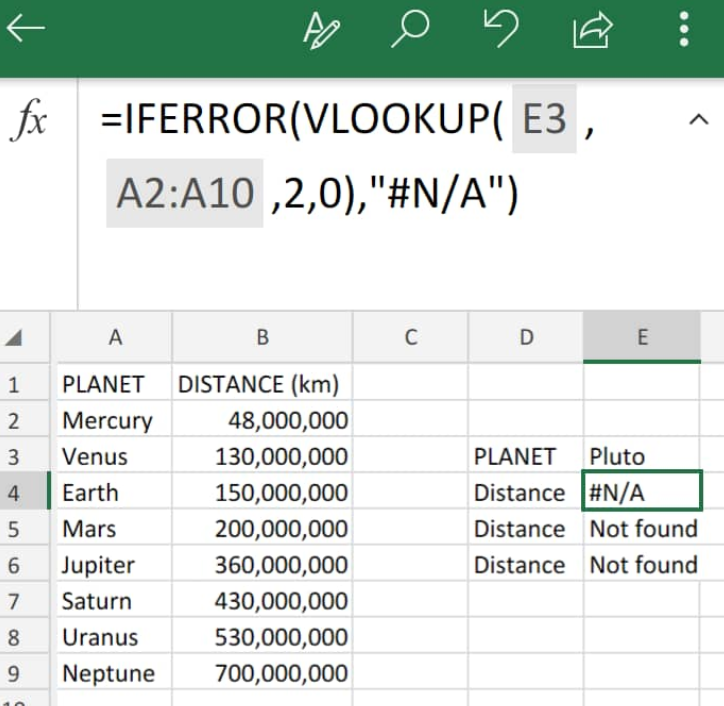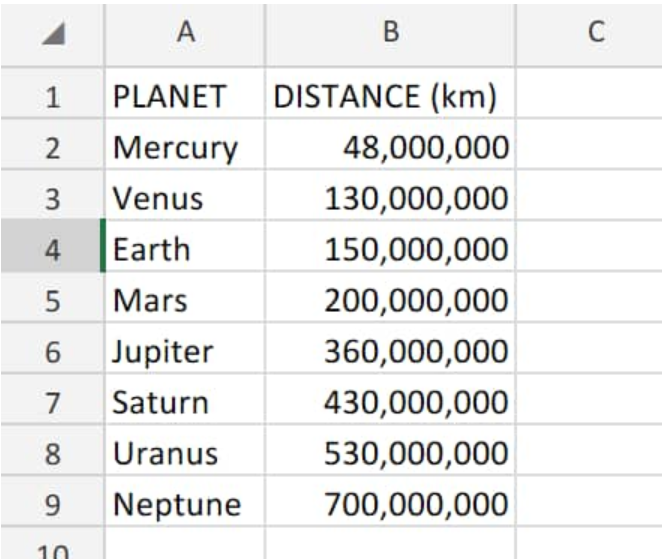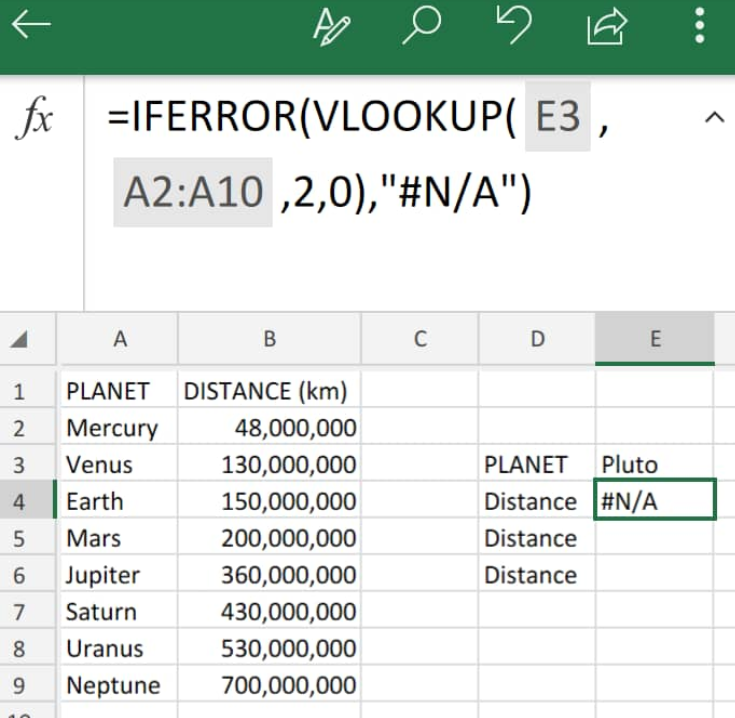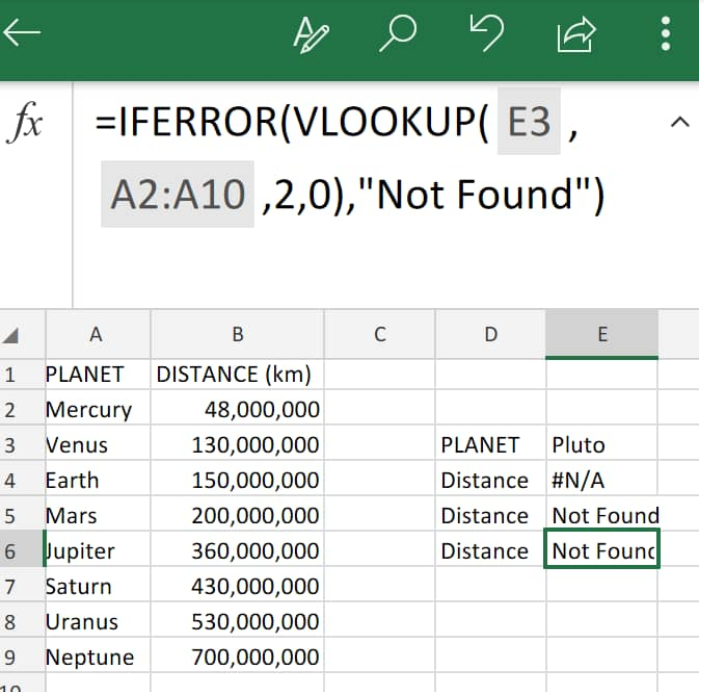Get instant live expert help with Excel or Google Sheets“My Excelchat expert helped me in less than 20 minutes, saving me what would have been 5 hours of work!”

#### Post your problem and you’ll get expert help in seconds.

Your message must be at least 40 characters
Our professional experts are available now. Your privacy is guaranteed.

# How to troubleshoot VLOOKUP issues in Excel – Excelchat

Excel Vlookup returns na error value when it fails to locate a match to our supplied lookup_value. A limitation of the VLOOKUP function is that it is only able to look for values that are on the extreme left column of a worktable array. If our lookup_value isn’t inside the first column, we will get a #N/A error in Excel.Figure 1. VLOOKUP Errors in Excel

## Generic Formula

`=IFERROR(VLOOKUP(lookup_value,table,2,FALSE),"text")`

We can manage the Excel #N/A error value that VLOOKUP returns whenever it can’t find a specified value; the IFERROR function can be used to catch the VLOOKUP error and then return any value we like.

## How to Fix VLOOKUP #N/A Errors in Excel

When VLOOKUP cannot find a value inside a lookup range, it returns the Excel #n/a error value. The IFERROR function will allow us to block such errors and input our own custom value to be returned whenever there’s an error.

We can do this with the following straightforward steps;

1. We can start by arranging our data values in our worksheet:Figure 2. Data Values for VLOOKUP in Excel

Our goal here is for VLOOKUP to return the value “Not Found” when it cannot find a match.

1. The formula for cell E4 in our worksheet example below is as follows;
`=IFERROR(VLOOKUP(E3,A2:A10,2,0),”N/A”)`Figure 3. VLOOKUP N/A in Excel

If VLOOKUP returns our value normally, then there’s no error and our looked up value gets returned. But If VLOOKUP returns n/a error value, IFERROR will kick-in and return the value we supply.

1. The formula for cell E6 in our worksheet example below is as follows;
`=IFERROR(VLOOKUP(E3,A2:A10,2,0),”Not Found”)`Figure 4. Fixing VLOOKUP Error in Excel

If we have a lookup_value in cell A2 and lookup values inside a range on our worksheet, and we want VLOOKUP to return a blank when our lookup_value is not found, we can use: “”

## Instant Connection to an Excel Expert

Most of the time, the problem you will need to solve will be more complex than a simple application of a formula or function. If you want to save hours of research and frustration, try our live Excelchat service! Our Excel Experts are available 24/7 to answer any Excel question you may have. We guarantee a connection within 30 seconds and a customized solution within 20 minutes.